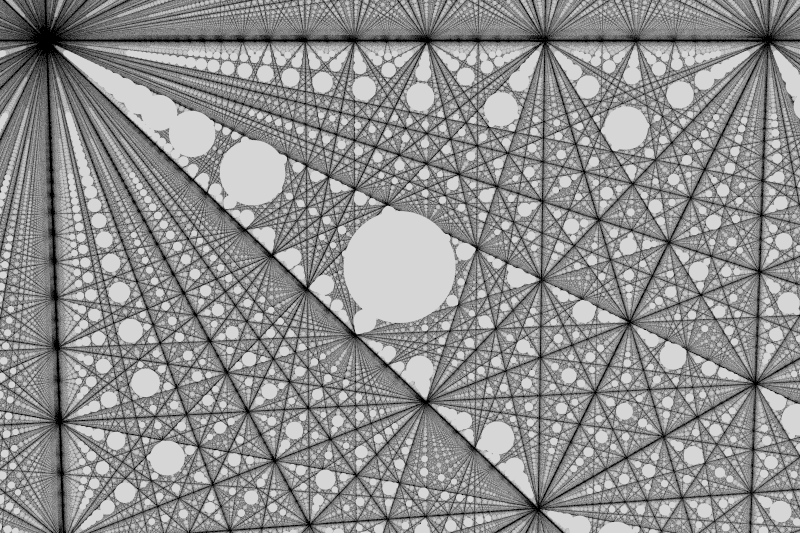# GLSL and Rust modulo operator

2020-10-09

I recently was playing around with raymarching and SDFs, and I tried to implement something to have infinite repetition using the modulo operator.

As it turns out, the `%` operator in Rust is not actually the modulo, but the remainder. As shown in this question, the difference between the two appears with negative numbers:

``````-21 modulus 4 => 3
-21 remainder 4 => -1
``````

Instead, GLSL uses the actual modulo, computed as `x - y * floor(x/y)` 1.

The fix is simple, we just have to create a function that computes the modulo using the formula above. In my case, I implemented it like this in my small math library for vectors:

``````pub fn modulo(&self, other: Vec3) -> Vec3 {
fn modulo(a: f32, b: f32) -> f32 {
a - (b * (a / b).floor())
}

Vec3 {
x: modulo(self.x, other.x),
y: modulo(self.y, other.y),
z: modulo(self.z, other.z),
}
}
``````

And here is the result with infinite white spheres: